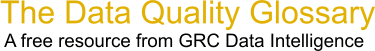Choose Index below for a list of all words and phrases defined in this glossary.

# Rolled Throughput Yield (RTY)

index | Index

## Rolled Throughput Yield (RTY) - definitions

Rolled Throughput Yield - RTY - Rolled Throughput Yield (RTY) is the probability that a single unit can pass through a series of process steps free of defects.

Next we will turn our attention to a Rolled Throughput Yield example. If you will remember, the First Time Yield calculation we did (FTY) considered only what went into a process step and what went out. Rolled Throughput Yield adds the consideration of rework. Using the previous example:

```   Process A = 100 units in and 90 out Process B = 90 in and 80 out Process C = 80 in and 75 out Process D = 75 in and 70 out.
```

If in order to get the yield out of each step we had to do some rework (which we probably did) then it really looks more like this:

```   Process A = 100 units, 10 scrapped and 5 reworked to get the 90. The calculation becomes [100-(10+5)]/100 = 85/100 = .85 This is the true yield when you consider rework and scrap.
Process B = 90 units in, 10 scrapped and 7 reworked to get the 80. [90-(10+7)]/90 = .81
Process C = 80 units in, 5 scrapped and 3 reworked to get the 75. [80-(5+3)]/80 = .9
Process D = 75 units in, 5 scrapped and 10 reworked to get the 70. [75-(5+10)]/75 = .8
```

Now to get the true Rolled Throughput Yield (Considering BOTH scrap and the rework necessary to attain what we thought was first time throughput yield) we find that the true yield has gone down significantly:

.85.81.9*.8 = .49572 or Rounded to the nearest digit, 50% yield. A substantially worse and substantially truer measurement of the process capability. An Assumption is made in the preceeding example that there are no spilled opportunities after each process step.

[Category=Data Quality ]

Source: iSixSigma, 21 February 2011 07:56:49, http://www.isixsigma.com/index.php?option=com_glossaryThese advertisers support this free service

Rolled Throughput Yield - Probability that a product will pass through the entire process without rework and defects. It is the product of the First Time Through (FTT) of all process steps.

[Category=Quality ]

Source: The Quality Portal, 22 April 2011 08:23:20, http://thequalityportal.com/glossary/l.htmData Quality Glossary.  A free resource from GRC Data Intelligence. For comments, questions or feedback: dqglossary@grcdi.nl